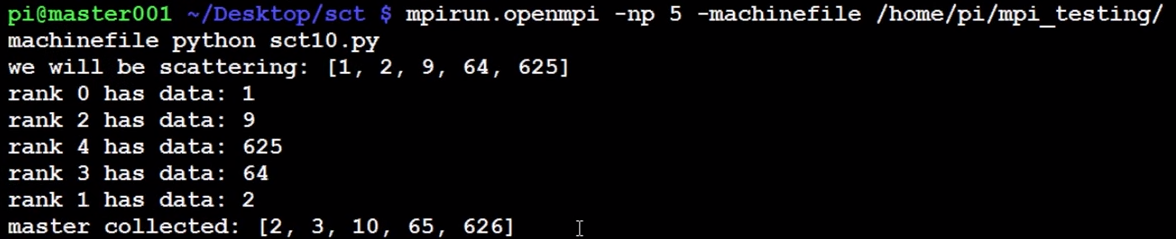## Gather command with MPI, mpi4py, and Python

### Scatter and Gather with MPI using MPI4py and Python

In this mpi4py tutorial, we're going to cover the gather command with MPI. The idea of gather is basically the opposite of scatter. Gather will be initiated by the master node and it will gather up all of the elements from the worker nodes.

We'll use almost an identical script as before with a few small changes. Let's say we scatter a bunch of data to the nodes, those nodes perform an operation on that data, and then we want the master node to collect the results. Here's how we'd do it:

```from mpi4py import MPI

comm = MPI.COMM_WORLD
size = comm.Get_size()
rank = comm.Get_rank()

if rank == 0:
data = [(x+1)**x for x in range(size)]
print 'we will be scattering:',data
else:
data = None

data = comm.scatter(data, root=0)
data += 1
print 'rank',rank,'has data:',data

newData = comm.gather(data,root=0)

if rank == 0:
print 'master:',newData
```

Here, all of the nodes are modifying the data variable. This data += 1 is our really intense operation that we want the nodes to perform in parallel! Next, we specify the gather command.

Gather works by specifying what we're gathering, and where the data will go (root), which we're saying is to processor 0.

This will show that the data was dispersed, the operation was performed, and the new data was correctly gathered back up.

`mpirun.openmpi -np 5 -machinefile /home/pi/mpi_testing/machinefile python ~/Desktop/sct/sct10.py`There exists 7 quiz/question(s) for this tutorial. for access to these, video downloads, and no ads.

That's all for this specific series. For more tutorials, head to the• Build a Supercomputer with Raspberry Pis

• Intro

• Supplies

• Installing Operating System

• Downloading and installing MPI

• Testing Supercomputer

• MPI with MPI4py Introduction

• Installing mpi4py for use with Python and MPI

• First basic MPI script with mpi4py

• Using conditional, Python, statements alongside MPI commands example

• Getting network processor size with the size command

• Sending and Receiving data using send and recv commands with MPI

• Dynamically sending messages to and from processors with MPI and mpi4py

• Message and data tagging for send and recv MPI commands tutorial

• MPI broadcasting tutorial with Python, mpi4py, and bcast

• Scatter with MPI tutorial with mpi4py

• Gather command with MPI, mpi4py, and Python